# Blood relation for IBPS, SBI, RBI, RRBs: Quiz 5

## Blood relation for IBPS, SBI, RBI, RRBs: Quiz 5

Blood relation plays a significant role in Reasoning Ability Section of banking exams such as IBPS, SBI and RBI PO and Clerk. You will get at least 2-3 questions from Blood relation in one of IBPS, SBI and RBI PO & clerk prelims and Mains exam. So, aspirants should focus on Blood relation questions in detail. Here, we are providing you with the Blood relation questions quiz with the detailed solution so that you can easily prepare for Blood relation questions. We are providing here all-important latest pattern-based questions and Previous Year Questions of Blood relation of various Government Exam like IBPS, SBI, and RBI PO and Clerk exam. This Blood relation quiz we are providing is free. Attempt this Blood relation quiz to practice important questions with answers and solutions. And score better in IBPS, SBI and RBI PO and Clerk exam.

Blood relation Quiz to improve your Reasoning Ability for SBI Po & SBI clerk exam IBPS PO Reasoning, IBPS Clerk Reasoning, IBPS RRB Reasoning, LIC AAO, LIC Assistant and another competitive exam.

All the given members belong to the same the family. J is the brother of L. J is the only son of R. W is the father-in-law of L. D is the maternal grandfather of P, who is a male. Q is the only son of W. W is the grandfather of N and C is the daughter of N.

1. How L is related to C?

(a) Mother

(b) Son

(c) Brother

(d) Father

(e) None of these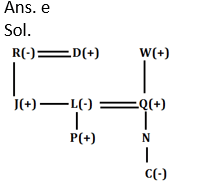1. How is P related to N?

(a) Mother

(b) Son

(c) Brother

(d) Father

(e) None of theseDirections (3-5): Study the following information carefully and answer the questions given below:

J is the mother of L who is married to P. R is the only son of P. A is the grandmother of R. V is the only sister of L. W is married to V. H is the only child of V. D is the brother-in-law of P. J has only two children.

1. How is D related to A?

(a) Mother

(b) Son-in-law

(c) Brother-in-law

(d) Father

(e) None of these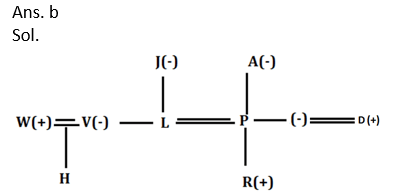1. How is H related to R?

(a) Mother

(b) Son

(c) Brother

(d) Cousin

(e) None of these1. How is W related to L?

(a) Mother

(b) Son-in-law

(c) Brother-in-law

(d) Father

(e) None of theseDirections (6-8): Study the following information carefully and answer the questions which follow–

‘K @ R’ means ‘K is wife of R’

‘K # R’ means ‘K is son of R’

‘K \$ R’ means ‘K is father of R’

‘K % R’ means ‘K is sister of R’1. Which of the following expression represents the relationship ‘T is mother of N’?

(a) T @ R \$ P % N

(b) T @ R # P \$ N

(c) T % R \$ P @ N

(d) T \$ R % P \$ N

(e) T # R % P @ N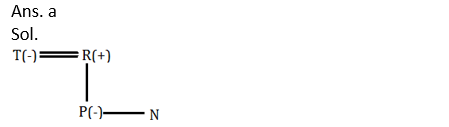1. In expressions ‘A % B # C \$ D’ how is B related to D?

(a) brother-in-law

(b) sister

(c) brother

(d) cousin

(e) None of these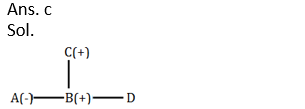1. Which of the following expression represents the relationship ‘M is brother of G’?

(a) G # T \$ M

(b) G % T \$ M

(c) M % G \$ T

(d) G % M \$ T

(e) M # T % G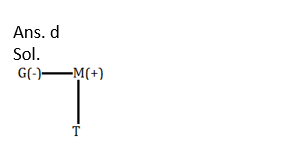Directions (9-10): Following questions are based on the information given below.

‘P × Q’ means ‘P is Son of Q’

‘P – Q’ means ‘P is sister of Q’

‘P + Q’ means ‘P is mother of Q’

‘P ÷ Q’ means ‘P is the brother of Q’

1. In the expression R ÷ Q + M ÷ N × G, how is N related to R?

(a) Granddaughter

(b) Son

(c) Niece

(d) Granddaughter or Grandson

(e) None of these1. In which of the following expression Q is wife of Z?

(a) A–M÷Q+N×Z

(b) A–Q÷M+N×Z

(c) Q–M÷A+N×Z

(d) Q–R÷A÷N×Z

(e) None of these#### Aatma Nirbhar Reasoning Topic Wise Online Test Series

Recommended PDF’s for :

#### Most important PDF’s for Bank, SSC, Railway and Other Government Exam : Download PDF Now

AATMA-NIRBHAR Series- Static GK/Awareness Practice Ebook PDF Get PDF here
The Banking Awareness 500 MCQs E-book| Bilingual (Hindi + English) Get PDF here
AATMA-NIRBHAR Series- Banking Awareness Practice Ebook PDF Get PDF here
Computer Awareness Capsule 2.O Get PDF here
AATMA-NIRBHAR Series Quantitative Aptitude Topic-Wise PDF Get PDF here
AATMA-NIRBHAR Series Reasoning Topic-Wise PDF Get PDF Here
Memory Based Puzzle E-book | 2016-19 Exams Covered Get PDF here
Caselet Data Interpretation 200 Questions Get PDF here
Puzzle & Seating Arrangement E-Book for BANK PO MAINS (Vol-1) Get PDF here
ARITHMETIC DATA INTERPRETATION 2.O E-book Get PDF here
3